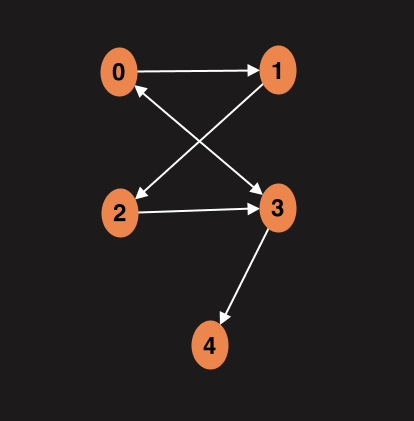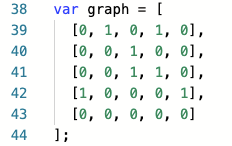1629011520

# JavaScript Algorithms and Data Structures: Trees - Breadth-First Search

Breadth-first search (BFS) is an algorithm for traversing or searching tree or graph data structures. It starts at the tree root (or some arbitrary node of a graph, sometimes referred to as a 'search key') and explores the neighbor nodes first, before moving to the next level neighbors.## Pseudocode

``````BFS(root)
Pre: root is the node of the BST
Post: the nodes in the BST have been visited in breadth first order
q ← queue
while root = ø
yield root.value
if root.left = ø
q.enqueue(root.left)
end if
if root.right = ø
q.enqueue(root.right)
end if
if !q.isEmpty()
root ← q.dequeue()
else
root ← ø
end if
end while
end BFS
``````

## References

The Original Article can be found on https://github.com

#javascript #algorithms #datastructures #trees

## Buddha Community1596737580

## Graphs Data Structure: Breadth First Search

A week ago we learned about graph data structure. Today we will talk about how we can work with graphs. We will try to find distances between two nodes in a graph. This is one of the main uses of graphs and it’s called graph traversal. There are two main graph algorithms Breadth First Search (BFS) and Depth First Search (DFS) and today we will talk about BFS.

This is how our graph looks like:In our example we will work with an adjacency matrix. This is how matrix represents graph above:We will start with an input node, then visit all its neighbors which is one edge away. And then visit all their neighbors. Point is to determine how close the node is to the root node.

Function which we will write in a moment will return an object with key value pairs where key will represent node and value how far this node is from the root.

First we will loop over the adjacency matrix (2D array), create as many key value pairs as many nodes we have on the graph. Initially we will assign distance to the infinity which represents lack of connection between the nodes.

#graph #data-structures #breadth-first-search #javascript #algorithms #algorithms1620466520

If you accumulate data on which you base your decision-making as an organization, you should probably think about your data architecture and possible best practices.

If you accumulate data on which you base your decision-making as an organization, you most probably need to think about your data architecture and consider possible best practices. Gaining a competitive edge, remaining customer-centric to the greatest extent possible, and streamlining processes to get on-the-button outcomes can all be traced back to an organization’s capacity to build a future-ready data architecture.

In what follows, we offer a short overview of the overarching capabilities of data architecture. These include user-centricity, elasticity, robustness, and the capacity to ensure the seamless flow of data at all times. Added to these are automation enablement, plus security and data governance considerations. These points from our checklist for what we perceive to be an anticipatory analytics ecosystem.

#big data #data science #big data analytics #data analysis #data architecture #data transformation #data platform #data strategy #cloud data platform #data acquisition1623066480

## JavaScript Data Structures and Algorithms (Search Algorithms, Part 1)

Hello! Today I would like to review what I’ve learned about search algorithms and the importance of each one in computer programming. I’ve never been great at writing preambles, so without further ado, let’s get started.

It’s safe to say we’ve all used some kind of search engine when browsing the web. Whether it’s Google, Bing, DuckDuckGo, etc., we have experienced the benefits of their search algorithms, individually and as a society. But how do search engines conduct these searches for optimized results from around the web? And how do they determine how results get sorted? The answer is more complicated than fundamental algorithms, though those still exist at the core of these search engine behemoths.

## Linear Search

As I mentioned, Google’s search algorithms are more than simple lookup() methods but are still used at the most rudimentary level. One thing I can say confidently is that this first algorithm is not how Google executes searches. **_Linear search _**algorithms search a sorted structure, such as an array or a binary search tree, to find a match to the desired value. For example, if I had declared a sorted array as [0,1,2,3,4,5,6], and I ran a linear search for the value 6, our search algorithm would start the search at , then visit every index in the array, comparing each index’s value with our target value, until a match is found.

Linear Search is arguably the most rudimentary of search algorithms. But like every other algorithm, trade-offs exist when implementing this as a solution. This search algorithm would not be great in the use-case of an array with 1,000,000 indices to search through for a value, given its time complexity. You see, this search algorithm has a potential time complexity of O(n), or linear time complexity. The time complexity of this algorithm directly correlates to the size of the data structure (An array with one index can run a search in O(1) time, two indices in 0(2 + n) time, simplified to O(n), and so on). Unfortunately, this also means an array with 1,000,000 indices would execute a linear search for a value at index 300,000 in O(300,000 + n) time or, simplified, O(n) time. We needn’t worry about having to conduct linear searches through large amounts of data; there are, indeed, more optimal solutions available.

## Binary Search

Binary searches, named after the trees they are programmed to search, search arrays in order, given that they are sorted. Binary searches work best when using a sorted array to build a binary search tree since we can create them effectively. To begin, the root node is defined as the median value of a sorted array. Going back to the previous array [1, 2, 3, 4, 5, 6, 7], this can be used to build a binary tree, which is more comprehensible for understanding and writing search algorithms. Does this look familiar to you?

This median key’s value gets compared to the search’s value to see if it is greater than or less than the target. Based on this comparison, a binary search algorithm will then search either array’s values to the left of the median if less than the median, or the right if greater. By now I’m hoping you remember that the lookup() method in a binary search tree works in this manner. The median value of a sorted array can be compared to the root node of a binary search tree, and lookup() for both require that the data structure is sorted. And, like with tree traversal, a binary search must visit every value in a sequence until a match is found. In a binary search tree with nodes valued [1,2,3,5,10,15,25,30,40,45,50], we would build a binary search tree using 15 as the tree’s root node, adding key values with lower values than the search value to the left, and ones with greater values to the right. If we were searching for a node with a value of 45, the algorithm would traverse in this order: root, left-child, right-child. An array representation of the nodes traversed for this would look like this: [15,25,40].

lookup(), as implemented in a binary search tree.

That’s all for now! In the next post, I will go over two more search algorithms: breadth-first search, and depth-first search, and how they are implemented in both trees and graphs. Be sure to check out https://visualgo.net for powerful visualizations of data structures and their algorithms, as well as a step-by-step breakdown of each of the latter. It’s probably the most useful learning aid I’ve used when learning.

#data-structures #trees #algorithms #javascript1626268740

## Depth First Search (DFS) in Data Structure

In the last article, we learned about graphs in data structures. Graphs are one of the efficient ways that are used to model daily life problems and find an optimal solution. In this article, we will learn about traversing techniques for the graph and their implementation

#### Depth First Search

DFS is a recursive traversal algorithm for searching all the vertices of a graph or tree data structure. It starts from the first node of graph G and then goes to further vertices until the goal vertex is reached.

• DFS uses stack as its backend data structure
• edges that lead to an unvisited node are called discovery edges while the edges that lead to an already visited node are called block edges.

#### DFS procedure

DFS implementation categorizes the vertices in the graphs into two categories:

• Visited
• Not visited

The major objective is to visit each node and keep marking them as “visited” without making any cycle.

#### Steps for DFS algorithms:

1. Start by pushing starting vertex of the graph into the stack

2. Pop the top item of the stack and add it to the visited list

3. Create the adjacency list for that vertex. Add the non-visited nodes in the list to the top of the stack

4. Keep repeating steps 2 and 3 until the stack is empty

#### Depth First Search Algorithm

• Step 1: STATUS = 1 for each node in Graph G
• Step 2: Push the starting node A in the stack. set its STATUS = 2
• Step 3: Repeat Steps 4 and 5 until STACK is empty
• Step 4: Pop the top node N from the stack. Process it and set its STATUS = 3
• Step 5: Push all the neighbors of N with STATUS =1 into the stack and set their STATUS = 2
• [END OF LOOP]
• Step 6: stop

#data structure tutorials #applications of depth first search #depth first search #data structure1629011520

## JavaScript Algorithms and Data Structures: Trees - Breadth-First Search

Breadth-first search (BFS) is an algorithm for traversing or searching tree or graph data structures. It starts at the tree root (or some arbitrary node of a graph, sometimes referred to as a 'search key') and explores the neighbor nodes first, before moving to the next level neighbors.## Pseudocode

``````BFS(root)
Pre: root is the node of the BST
Post: the nodes in the BST have been visited in breadth first order
q ← queue
while root = ø
yield root.value
if root.left = ø
q.enqueue(root.left)
end if
if root.right = ø
q.enqueue(root.right)
end if
if !q.isEmpty()
root ← q.dequeue()
else
root ← ø
end if
end while
end BFS
``````

## References

The Original Article can be found on https://github.com

#javascript #algorithms #datastructures #trees